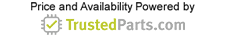Industrial Electronics

# Anadyne’s Next-Gen Detector Log Video Amplifier Foils CW Jamming Threats

28 April 2017

Many applications measure physical quantities over a wide dynamic range. These applications use logarithmic amplifiers (log amps) to match a transmitter's dynamic output to the linear input range of a signal gauge. Figure 1 shows a typical signal being sensed by a sensor. The sensor has an output range of seven decades; if we want to measure the output of this sensor directly we would need a meter with at least seven decades scale. However if the input of the sensor is passed through a log amplifier, as shown in the figure, we can measure the signal with a gauge of one decade scale only.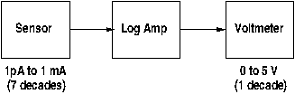Figure 1. Sensor system.

Due to the fact that a log amp compresses the signal, we may think that some information will be lost, but this is not the case with log amps. The log amp compresses intelligently. To understand this, let’s consider an example.

Suppose we need to make some “measurement,” and let’s assume that the measuring device is an analog-to-digital (A/D) converter. We have some source of pulses whose pulse heights, x, represents the quantity we are interested in measuring. These pulses go through a linear amplifier whose output, y, goes into the A/D. Let’s assume that we want to measure each pulse height to 10% and the dynamic range of the pulses of interest is 105. Further assume that the only error of significance is the unavoidable quantization error,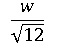where w is the width of the bin containing y. We will examine the results with both the linear transfer and then with a log conversion amplifier.

If we use a linear transfer, then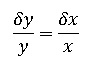and the percentage error in y is the same as that in the quantity we want to measure. Clearly the largest percentage error will occur for the smallest value of y, since δy = w/√12, and for a linear system, w is constant. The question we need to ask is how wide the A/D we need should be (how many bits). If we use N bits, then we require,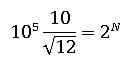then,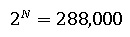therefore,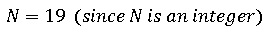So, we need a high-speed 19 bits A/D! Tough for a high-speed A/D.

Now let us suppose that we used a logarithmic converter. The full range is 105 or 100 dB. With a log conversion, dy = dx/x, (differentiating the log). The question is just how small we have to make the bins.

With an allowed error of 10%, (0.82 dB), we require bin widths of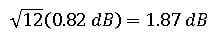So we need 53 bins to cover the dynamic range of 100 dB. Therefore we need only use a 6 bit A/D!

How did we achieve such a remarkable reduction? Have we lost anything? The answer to the second question is no, we have not. Well if that’s the case, what were all those thousands of bins doing in the linear case? The answer is very simple. For the smallest pulse, we got 10% accuracy; however, for pulses that were close to the largest, we got a ridiculous accuracy: 10-4. This huge number of bins is actually a nuisance as the occupancy is extremely low with so many bins. What the log amp does is to keep the error constant, as the gain of a log converter falls inversely with the pulse height. This is precisely what is needed to keep δy/y constant, since δy is constant. Thus, the log converter packs data in the most efficient manner for the cases where one wants constant error in dB.

Log amps of the past were built with hybrid techniques. Today's technology enables the integration of the logging circuit and additional support functions, such as voltage references, low offset control, large bandwidth and uncommitted operational amplifiers.

The term logarithmic amplifier, or simply log amp, is really a misnomer. The true operation of the device is to convert an input quantity (such as voltage) into an output value proportional to the logarithm of the input, so a more apt name for the device would be ‘logarithmic converter.’ In particular, the log amp will convert an input signal to its equivalent logarithmic value (multiplied by a constant, in many cases.) The conversion is a non-linear operation and the device is a non-linear electronic device. This implies that many of the standard circuit concepts of typical linear circuits do not apply to log amps. For example, the gain of a log amp is inversely proportional to the input amplitude, so it approaches infinity as the input signal tends to zero. This is clearly not something any amp can do, so one can only make an amp with a logarithmic transfer over a finite range that does not include zero

When trying to maximize the dynamic range, it is advantageous to start with the smallest input possible or the top of end of the range will be unnecessarily large, which creates problems. Thus, the amplifier requires very large gain for small inputs, necessitating excellent temperature stability. The non-linear transfer curve exacerbates the problem, as a change in input offset not only shifts the output, but ruins the logarithmic response for values of the input that are not much larger than the offset.

Basic log amp

The basic logarithmic amplifier is a simple op amp with a diode feedback, as is shown figure 2.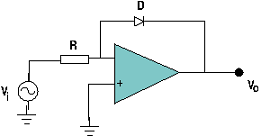Diode log amp.

Actually, it need not be a diode; any device with an exponential relationship between the voltage across it and the current through it will give an output voltage that is logarithmically proportional to the input signal strength. A diode is not actually a very good choice as non-ideality sets in at a low current, and then the Ebers Moll equation is not obeyed, so the logarithmic transfer is not accurate. It is better to use a diode-connected transistor as they follow the Ebers Moll relationship over a wider range.

In addition, there are two weaknesses with this basic type. Firstly the dynamic impedance of the feedback element is very high for small inputs, which slow the amplifier down for low amplitude inputs. Secondly, the temperature compensation is difficult.

In practice these are not used if bandwidths in excess of 1 Mhz are needed. For a description of how a typical log video amplifier is actually made, see the Anadyne Inc. website’s technical section, or perform an Internet search for: “Richard Hughes, logarithmic amplifiers.” Note that although log amps made as described in the references above deviate from an ideal log curve in the range of interest, by about 1/3 dB, if the amps are stable over temperature, or are running at fixed temperature, they can be calibrated to much higher accuracy.

Types of specialized log amplifiers

There are three main types of logarithmic amplifiers: (1) detector log video amplifier (DLVA), (2) successive detection log video amplifier (SDLVA or SDLA), and (3) true log amplifier (TLA).

Detector Log Video Amplifier (DLVA)

A detector log video amplifier (DLVA) is technically any setup that utilizes a transducer to convert the physical effect being measured to a voltage or current that is then passed through an LVA to produce an output that is a logarithmically proportional to the magnitude of input of the effect. In practice the term is usually applied to a system that detects the envelope of an RF signal by means of a diode detector. This is a circuit that provides an output voltage proportional to the power level of the RF input signal. The DLVA can compress a wide dynamic range of RF power levels into a narrow range of video voltage. Henceforth we will be referring to this type of DLVA in the discussion that follows.

Structurally, a DLVA is basically a detector followed by a LVA. Generally, the detector is a tunnel diode (with zero bias) or a Schottky barrier diode. The type of detector used depends on the specifications of the LVA in order to get a maximum transfer of power between the two stages. If a Schottky diode is used then an LVA with high input impedance is required, whereas if a tunnel diode is the detector, a low input impedance of the LVA is needed.

Extended range DLVA (ERDLVA)

Extended range DLVAs may use more than one detector to achieve a larger dynamic range.

As we have established, the dynamic range of a DLVA is limited by the sensitivity of the detector. A typical square law diode detector will have a TSS of around -48 dBm with a 20 Mhz video bandwidth. The RF noise floor with this video bandwidth is around -100 dBm. Thus, sensitivity can be improved if we use a linear pre-amplifier (gain block) in front of the detector. Unfortunately, the detector would saturate at very low power, but this came be overcome by splitting the RF and putting a second detector without RF amplification, often attenuation in fact, in parallel with the first detector and limiting its input at the power when the second detector becomes sensitive. One can add a third detector if need be and dynamic ranges in excess of 100 dBm can be achieved in this manner.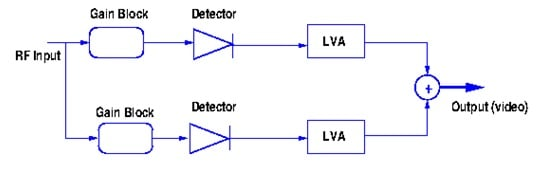Figure 3. Extended range DLVA.

Successive Detection Log Video Amplifier

The successive detection log amplifier (SDLA) and the successive detection log video amplifier (SDLVA) are a variation of the ERDLVA. Also called demodulating log amps, both compress and demodulate RF signals, yielding the logarithm of the rectified signal's envelope. Demodulating log amps are prevalent in RF transceiver applications where the received RF signal strength is measured to control transmitter output power.

The operation of this system can be explained as follows. As the amplitude of the input signal, RF in, is increased, each of the gain stages goes into compression. This compression starts at the output stage and progresses toward the input stage. The detector stages that are connected to the outputs of the gain stages and at the input of the first gain stage produce currents that are proportional to the signal voltages at these points. These currents, I0, I1, I2…IN, are summed simply by connecting the outputs of all the detectors in parallel. The sum of these output currents, IS = I0 + I1 + I2…IN, is logarithmically related to the input signal's magnitude. A typical block diagram of an SVDLVA is shown in figure 8.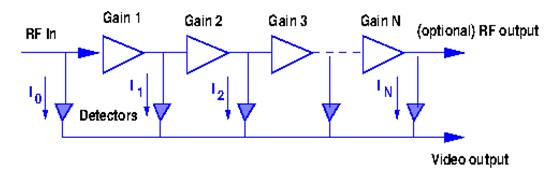Figure 4. Successive DLVA.

The typical dynamic range of each amplifier/detector stage is approximately 10 dB, so many such stages are needed to cover a large dynamic range. A SDLVA can also produce an optional second output representing a limited RF/IF signal, as seen in figure 8. This output is an amplified replica of the input signal.

Log Video or True Log Amplifier

The third class of log amp is known as the true log amplifier (TLA). A TLA accepts bipolar inputs and provides a compressed output voltage that preserves the polarity of the input. True log amps are sometimes used in radio IF stages and medical ultrasound receiver circuits for dynamic range compression.

CW Immune DLVAs

DLVAs are designed to detect power pulses, even in the presence of other RF signals, including CW RF signals. The ability to suppress CW RF waveforms is an important design parameter called CW immunity, and it is formally defined as the power range (generally in dBm) over which the CW signals will be rejected. For DLVA manufacturers and designers this is a difficult task. Few companies can claim to have the upper hand when manufacturing DLVAs.

Let's look at the jamming problem posed by CW signals. Suppose an RF diode detector is connected to a log amp. In addition, suppose that there is a CW signal with frequency WC, which we shall call the jamming signal, plus various other signals that may be pulses or CW. The jamming signal is detected by the diode which produces a DC offset into the input of the log amp. Unless the amplitude of another signal we want to detect is much larger than that of the jamming signal, it will not be measured correctly because the log amp is not linear and the effective gain could be substantially reduced by the CW jamming signal. With sufficient power, the jamming signal could cut the gain to such an extent that small signals would be invisible.

RF or microwaves signals are often detected using tunnel or Schottky diodes. The output voltage on the diode is linearly related to the input power at power less than about -20 dBm. If the RF power incident on the diode is increased to a value that is higher than the power of the output voltage, then the roll-off (sensitivity) is lost. The roll off begins at the output voltage where the diode I-V curve starts to depart from Ebers Moll behavior. The reason is the same: lack of mobility causes charge crowding and departure from Ebers Moll. Diode-connected transistors obey Ebers Moll over a far larger range than diodes. The reason is that the base is so thin that mobility is not as serious a problem. Of course, diode connected transistors tend to have a lower reverse breakdown voltage than diodes do, but this is not a serious problem here as the voltages, even at powers above 0 dBm, are relatively low.

The sensitivity of the log amp can be restored if a signal matching the amplitude of the jamming signal is fed into the opposite polarity input to the log amp. In practice the detector for a CW immune system is a Schottky and it is fed into the non-inverting input of the log amp, so for the system under discussion it should be put into the inverting input. Provided the log amp has sufficient common mode capability range, the output of the log amp will then be zero and the gain will be fully restored. The first step to restore sensitivity using this method is for the user to distinguish between CW and pulsed inputs. They usually assign a time, τ, to be the longest pulse duration; anything longer will be called CW. The time, τ, can vary from 200 ns to 1 ms, depending on the application. Figure 9 illustrates several important parameters.

DLVAs are just log amps with square law detectors providing the input. As we have seen, DLVAs can be DC or AC-coupled. The former are more versatile as they can measure any length pulse accurately and they do not have severe duty cycle limitations for unipolar inputs. Unfortunately, it is a lot more difficult to make a DC-coupled DLVA if it has to operate over a broad temperature range. The broad dynamic range requires that the start of the logging range should occur at a very small input voltage or the circuit would have to take very large inputs. Small input voltages imply that the amplifier has to have a large gain for small inputs to make them usable.

DC-coupled units use tunnel diode detectors. Schottky diodes are unsuitable due to the fact that it is difficult to compensate temperature-induced drifts from Schottkys. For DC-coupled units required to run over a substantial temperature range, the gain of the unit and the offset need to be temperature independent. The gain can be kept stable by using heavy feedback, but the offset drift is far more difficult to manage, particularly with small geometry transistors that do not match well. Log amps have several serial gains, and if one can use AC coupling between the gain stages, the offset drift ceases to be a problem. However, there is a real need for DC-coupled devices for the reasons mentioned above and these units invariably use tunnels in spite of the lower gain of the tunnels and their frail nature.

To evaluate and describe CW strippers we need to define some performance parameters. Figure 9 shows some of these parameters that are important when designing DLVA’s CW strippers.

• Attack time is the time that elapses from t = τ until the output is sufficiently low, so that the log amp measurement of any pulse that comes in is within some specified difference from the value that would have been measured for it with no CW present. It should be clear that the shorter the attack time, the better.
• Droop is the maximum change in output pulse height for a fixed input signal for the time interval t = 0 to t = τ. Note that making the droop small is desirable for those using the DLVA, but it makes the attack time very long. As an illustration, if we have 35 dB of CW to strip and we require 0.2 dB maximum droop, then the attack time will be about 35/.2τ = 175τ. The attack time needs to be low to eliminate dead time. It is important to limit the attack time to a time less than τ.
• Recovery time. This is the maximum time for the unit to return to the baseline after a pulse that is shorter than the longest desired pulse.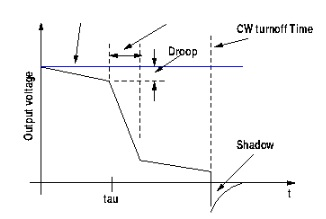Figure 5. Important Parameters for CW stripping.

Restorers

To strip undesirable CW signals in DLVA systems, an extra circuit called a restorer is added to the detector system. There are two basis classes: passive, which change the behavior of the circuit after t = τ, and active, which changes the behavior of the circuit when a signal remains above a threshold for a time greater than τ.

Some other problems to consider are the sensitivity loss of the diodes. Stripping large CW values is exceedingly difficult; as the CW signal rises, so do the currents and induced voltages and with this the diodes lose sensitivity once they begin to roll off.

Solving Key DLVA issues

There are many companies manufacturing logarithmic amplifiers, but each one produces DLVAs that do not solve one or more of the aforementioned problems.

Yet, Anadyne Inc., a small Silicon Valley-based RF company, has made unprecedented innovations in DLVAs. Their major achievements include:

1. Reduction of the attack times. Reducing the attack time to a constant, independent of the pulse duration, is really significant and makes it possible to beat the modulated CW attack. The remarkably short attack time achieved by Anadyne enables these units to overcome undesired new jamming techniques

2. Stripping more CW power than anyone else in a reasonable time. They have developed a method of stripping 40 dB of CW in less than 1 ms for any desired pulse length, which makes them independent of the pulse length, C!

3. CW suppression: The design of several manufacturing restorers to accomplish almost total CW suppression.

4. The improvement in duty cycle. While it is very difficult to achieve duty cycles above 50%, Anadyne's products routinely achieve duty cycles of 90% or more.Anadyne Inc.

PO Box 1344

Santa Cruz, CA 95061

831-438-4898